# E-PolyLearning

 21. Choose the figure which is different from the rest.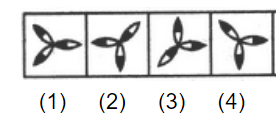a. 1 b. 2 c. 3 d. 4

 22. Choose the figure which is different from the rest.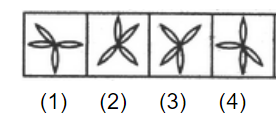a. 1 b. 2 c. 3 d. 4
 23. Choose the figure which is different from the rest.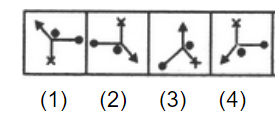a. 1 b. 2 c. 3 d. 4
 24. Choose the figure which is different from the rest.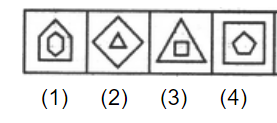a. 1 b. 2 c. 3 d. 4
 25. Choose the figure which is different from the rest.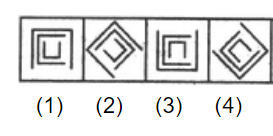a. 1 b. 2 c. 3 d. 4